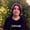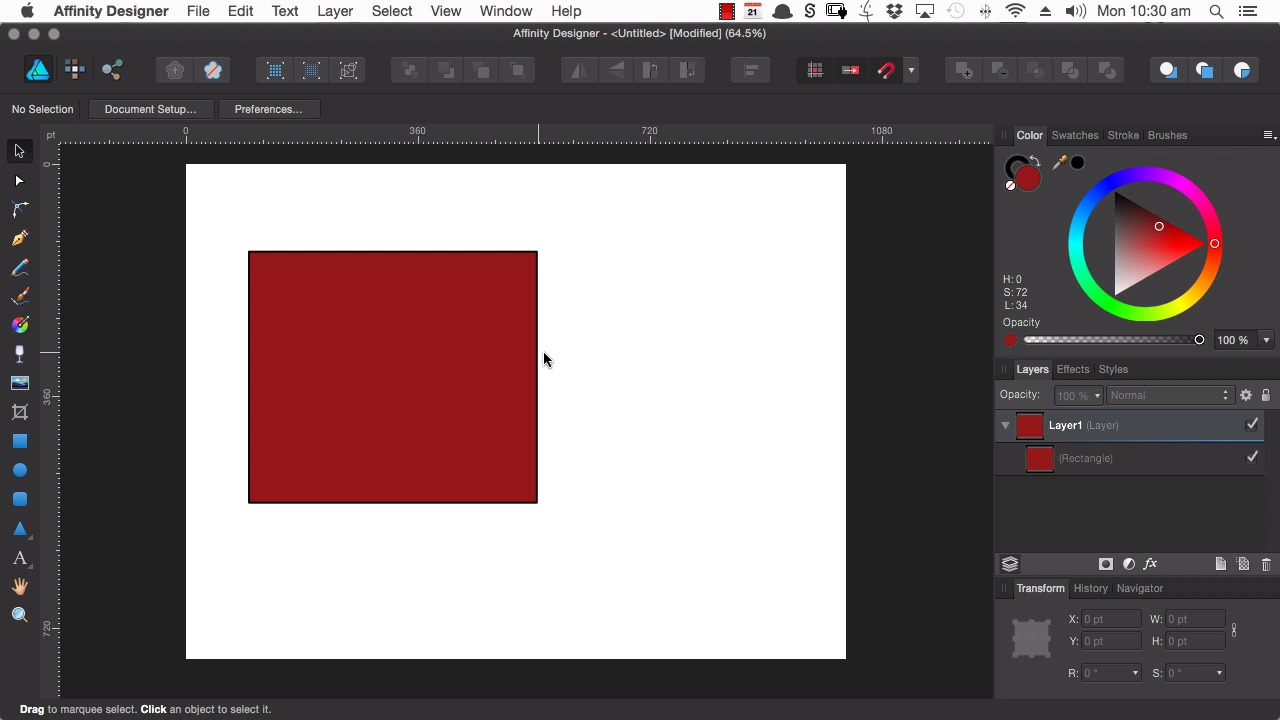Lessons: 35Length: 2.7 hours• Overview
• Transcript

# 6.1 Using Boolean Functions

Affinity Designer includes a wonderful set of Boolean functions for adding shapes together, subtracting, intersecting and more. Learn how to make use of them in this lesson.

## 10. Bonus Lessons5 lessons, 47:14

### 6.1 Using Boolean Functions

Hi, welcome back to Affinity Designer Quick Start. In this lesson, you're gonna learn about Affinity Designer's boolean functions. Boolean operations are a type of interaction between any two or more shapes. There are five different kinds of them, and you can see all five up in the top right corner of your interface here. All right, so let's grab the rectangle tool, and we'll draw out a couple of squares on the page, so that we can check out each one of these functions. In order to use Boolean functions, you'll need to make sure you have at least two shapes selected. Once you do you see that all of your boolean tools. Become highlighted so that they're available for use. All right now the first one will check out. Is the add tool and right now you can see now layers panel. We have two separate shapes. If we use this add tool that will combine those shapes into one. So you saw the outline disappear and now this is a single curve that we can edit accordingly. Now one purpose that this is particularly useful for is duplicating a shape so that you can have a symmetrical layouts. So, for example if we were to commit it for to create a shape. Like this. And we needed to reflect the shape. So that we had the exact same layout, on the other side. So to duplicate, and Flip that shape. Maybe over to here. And now we can combine these shapes. So now we have perfect symmetry. So the next boolean function that we will look at is subtract. So once again we will select the shapes. And then he'd subtract. You'll see that the icons he give you a pretty good idea of what this function is going to do so hit subtract. And you could see that this shape subtracted itself from this one. And if you have a look at the Layers palette again. This is the shape that's gonna be creating a cutout as the top shape. So let's say you wanted to create a sort of a scoop out in the middle here, you could draw out a circle, position it where you needed it to be for your scoop out. And then hit the subtract button. Next, let's have a look at intersecting. Draw out two squares. So intersecting is going to find the area in which these two shapes intersect. Select both of the shapes, and then hit intersect. Next up let's have a look at the divide boolean operation. So we'll just use some simple squares again. Now the divide operation is kind of similar to the one that we just used. It does find the intersecting shape here. But rather than are only giving you this shape and then deleting these extra areas it will divide these areas so that you end up with one, two, three spots. So now we have one, two, three separate shapes. And then finally we have the Combine operation, which is basically the opposite of the Intersect operation. So just draw out two more squares. Now once more this is going to find the area where these two shapes cross over, but it's going to delete it. So we'll have this shape and the shape remaining, with this overlapping area gone. So that's all five boolean functions in affinity design a say. They're very very useful you can use them in multiple different Y. S.. So just keep those in mind as you're going to remap new designs they can give you a lot of shortcuts and many things a lot quicker. In the next lesson we're going to look at another set of behaviors as far as shapes interacting with each other in infinity designer and this time we're going to be looking at masking and clipping where you take one shape and use it to obscure a pot of another shape. I'll see you then.

Back to the top